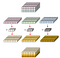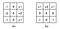# Depth-wise Convolution and Depth-wise Separable Convolution

Standard convolution layer of a neural network involve input*output*width*height parameters, where width and height are width and height of filter. For an input channel of 10 and output of 20 with 7*7 filter this will have 2800 parameters. Having so much parameters increases the chance of over-fitting. To avoid such scenarios, people have many a times looked around for different convolutions. Depth-wise convolution and depth-wise separable convolution fall into those categories.

## Depth-wise convolution

In this convolution, we apply a 2-d depth filter at each depth level of input tensor. Lets understand this through an example. Suppose our input tensor is 3* 8 *8 (input_channels*width* height). Filter is 3*3*3. In a standard convolution we would directly convolve in depth dimension as well (fig 1).

In depth-wise convolution, we use each filter channel only at one input channel. In the example, we have 3 channel filter and 3 channel image. What we do is — break the filter and image into three different channels and then convolve the corresponding image with corresponding channel and then stack them back (Fig 2)Fig 2. Depth-wise convolution. Filters and image have been broken into three different channels and then convolved separately and stacked thereafter

To produce same effect with normal convolution, what we need to do is- select a channel, make all the elements zero in the filter except that channel and then convolve. We will need three different filters — one for each channel. Although parameters are remaining same, this convolution gives you three output channels with only one 3-channel filter while, you would require three 3-channel filters if you would use normal convolution.

## Depth-wise Separable Convolution

This convolution originated from the idea that depth and spatial dimension of a filter can be separated- thus the name separable. Let us take the example of Sobel filter, used in image processing to detect edges. You can separate the height and width dimension of these filters. Gx filter (see fig 3) can be viewed as matrix product of [1 2 1] transpose with [-1 0 1]. We noticeFig 3. Sobel Filter. Gx for vertical edge, Gy for horzontal edge detection

that the filter had disguised itself. It shows it had 9 parameters but it has actually 6. This has been possible because of separation of its height and width dimensions. The same idea applied to separate depth dimension from horizontal (width*height) gives us depth-wise separable convolution whare we perform depth-wise convolution and after that we use a 1*1 filter to cover the depth dimension (fig 3).